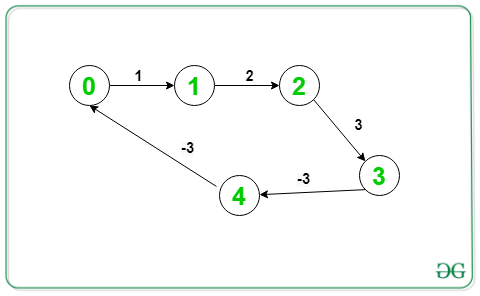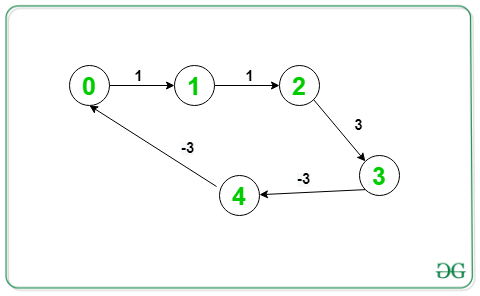# Print negative weight cycle in a Directed Graph

Given a weighted directed graph consisting of V vertices and E edges. The task is to print the cyclic path whose sum of weight is negative. If there is no such path present then print “-1”.

Input: V = 5, E = 5, Below is the graph:Output: 1 2 3 4 1
Explanation:
Given graph contains a negative cycle, (1->2->3->4->1)

Input: V = 5, E = 5, Below is the graph:Output: 0 1 2 3 4 0
Explanation:
Given graph contains a negative cycle, (0->1->2->3->4->0)

## Recommended: Please try your approach on {IDE} first, before moving on to the solution.

Approach: The idea is to use Bellman-Ford Algorithm which is used to detect a negative cycle or not. To print the negative cycles, perform the Nth iteration of Bellman-Ford and pick a vertex from any edge which is relaxed in this iteration. Using this vertex and its ancestors, the negative cycle can be printed. Below are the steps:

• Perform N-1 iterations of Bellman-Ford algorithm and relax each edge (u, v). Keep track of parent of each vertex and store in an array parent[].
• Now, do one more iteration and if no edge relaxation take place in this Nth iteration, then there is no cycle of negative weight exists in the graph.
• Otherwise take a variable C and store the vertex v from any edge (u, v), which is relaxed in the Nth iteration.
• Now, starting from the C vertex go towards its ancestors until a cycle is found and finally print it.
• This cycle will be the desired cycle of negative weight.

Below is the implementation of the above approach:

## CPP

 `// C++ program for the above approach ` `#include ` `using` `namespace` `std; ` ` `  `// Structure to represent a weighted ` `// edge in graph ` `struct` `Edge { ` `    ``int` `src, dest, weight; ` `}; ` ` `  `// Structure to represent a directed ` `// and weighted graph ` `struct` `Graph { ` ` `  `    ``// V -> Number of vertices, ` `    ``// E -> Number of edges ` `    ``int` `V, E; ` ` `  `    ``// Graph is represented as an ` `    ``// array of edges ` `    ``struct` `Edge* edge; ` `}; ` ` `  `// Creates a new graph with V vertices ` `// and E edges ` `struct` `Graph* createGraph(``int` `V, ``int` `E) ` `{ ` `    ``struct` `Graph* graph = ``new` `Graph; ` `    ``graph->V = V; ` `    ``graph->E = E; ` `    ``graph->edge = ``new` `Edge[graph->E]; ` `    ``return` `graph; ` `} ` ` `  `// Function runs Bellman-Ford algorithm ` `// and prints negative cycle(if present) ` `void` `NegCycleBellmanFord(``struct` `Graph* graph, ` `                         ``int` `src) ` `{ ` `    ``int` `V = graph->V; ` `    ``int` `E = graph->E; ` `    ``int` `dist[V]; ` `    ``int` `parent[V]; ` ` `  `    ``// Initialize distances from src ` `    ``// to all other vertices as INFINITE ` `    ``// and all parent as -1 ` `    ``for` `(``int` `i = 0; i < V; i++) { ` ` `  `        ``dist[i] = INT_MAX; ` `        ``parent[i] = -1; ` `    ``} ` `    ``dist[src] = 0; ` ` `  `    ``// Relax all edges |V| - 1 times. ` `    ``for` `(``int` `i = 1; i <= V - 1; i++) { ` `        ``for` `(``int` `j = 0; j < E; j++) { ` ` `  `            ``int` `u = graph->edge[j].src; ` `            ``int` `v = graph->edge[j].dest; ` `            ``int` `weight = graph->edge[j].weight; ` ` `  `            ``if` `(dist[u] != INT_MAX ` `                ``&& dist[u] + weight < dist[v]) { ` ` `  `                ``dist[v] = dist[u] + weight; ` `                ``parent[v] = u; ` `            ``} ` `        ``} ` `    ``} ` ` `  `    ``// Check for negative-weight cycles ` `    ``int` `C = -1; ` `    ``for` `(``int` `i = 0; i < E; i++) { ` ` `  `        ``int` `u = graph->edge[i].src; ` `        ``int` `v = graph->edge[i].dest; ` `        ``int` `weight = graph->edge[i].weight; ` ` `  `        ``if` `(dist[u] != INT_MAX ` `            ``&& dist[u] + weight < dist[v]) { ` ` `  `            ``// Store one of the vertex of ` `            ``// the negative weight cycle ` `            ``C = v; ` `            ``break``; ` `        ``} ` `    ``} ` ` `  `    ``if` `(C != -1) { ` ` `  `        ``for` `(``int` `i = 0; i < V; i++) ` `            ``C = parent[C]; ` ` `  `        ``// To store the cycle vertex ` `        ``vector<``int``> cycle; ` `        ``for` `(``int` `v = C;; v = parent[v]) { ` ` `  `            ``cycle.push_back(v); ` `            ``if` `(v == C ` `                ``&& cycle.size() > 1) ` `                ``break``; ` `        ``} ` ` `  `        ``// Reverse cycle[] ` `        ``reverse(cycle.begin(), cycle.end()); ` ` `  `        ``// Printing the negative cycle ` `        ``for` `(``int` `v : cycle) ` `            ``cout << v << ``' '``; ` `        ``cout << endl; ` `    ``} ` `    ``else` `        ``cout << ``"-1"` `<< endl; ` `} ` ` `  `// Driver Code ` `int` `main() ` `{ ` `    ``// Number of vertices in graph ` `    ``int` `V = 5; ` ` `  `    ``// Number of edges in graph ` `    ``int` `E = 5; ` ` `  `    ``struct` `Graph* graph = createGraph(V, E); ` ` `  `    ``// Given Graph ` `    ``graph->edge.src = 0; ` `    ``graph->edge.dest = 1; ` `    ``graph->edge.weight = 1; ` ` `  `    ``graph->edge.src = 1; ` `    ``graph->edge.dest = 2; ` `    ``graph->edge.weight = 2; ` ` `  `    ``graph->edge.src = 2; ` `    ``graph->edge.dest = 3; ` `    ``graph->edge.weight = 3; ` ` `  `    ``graph->edge.src = 3; ` `    ``graph->edge.dest = 4; ` `    ``graph->edge.weight = -3; ` ` `  `    ``graph->edge.src = 4; ` `    ``graph->edge.dest = 1; ` `    ``graph->edge.weight = -3; ` ` `  `    ``// Function Call ` `    ``NegCycleBellmanFord(graph, 0); ` ` `  `    ``return` `0; ` `} `

Output:

```1 2 3 4 1
```

Time Complexity: O(V*E)
Auxiliary Space: O(V)

Attention reader! Don’t stop learning now. Get hold of all the important DSA concepts with the DSA Self Paced Course at a student-friendly price and become industry ready.

My Personal Notes arrow_drop_upCheck out this Author's contributed articles.

If you like GeeksforGeeks and would like to contribute, you can also write an article using contribute.geeksforgeeks.org or mail your article to contribute@geeksforgeeks.org. See your article appearing on the GeeksforGeeks main page and help other Geeks.

Please Improve this article if you find anything incorrect by clicking on the "Improve Article" button below.